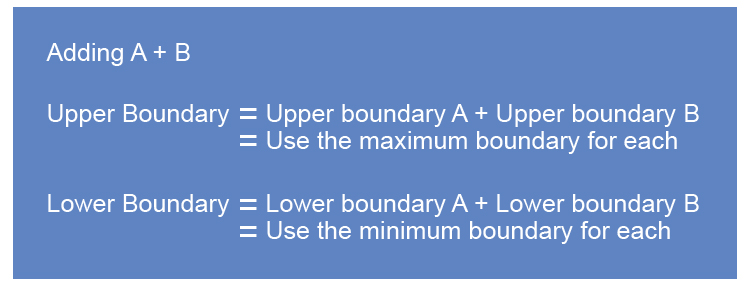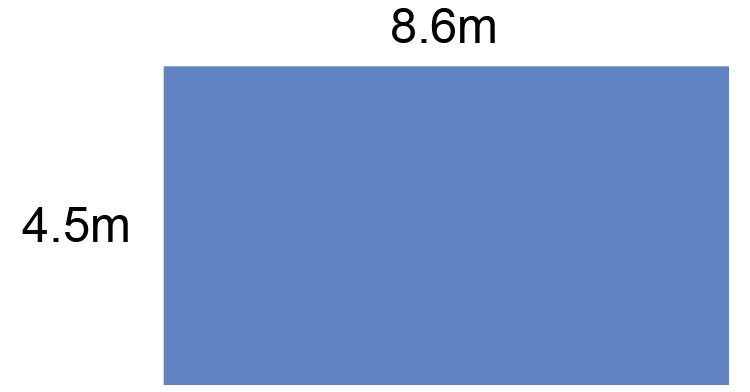# Upper and lower boundary addition

If you are looking to add two quantities and you need to know the possible limits upper boundary and lower boundary use logic:NOTE:

1. Upper boundary = Upper boundary A+ Upper boundary B

Adding the two largest possible upper boundaries will provide you with the biggest quantity possible.

Upper boundary = Big + Big = Largest amount

2. Lower boundary = Lower boundary A+ Lower boundary B

Adding the two smallest possible lower boundaries will provide you with the smallest quantity possible.

Lower boundary = Smallest + Smallest = Smallest amount

Example 1

Two pallets were stacked one above the other. The first was 120cm high and the second was 110cm high. You found out that the measurements were rounded to the nearest cm. What were the maximum and minimum heights of the stack?

The question is an addition question but the first thing you must do is find the maximum and minimum values of each number.

The upper and lower boundary of 120cm is

120cm to the nearest 1cm

12ul0 underline the last significant number.

12ul0\.0 look next door

0      5 or more raises the score. So 119.5 would raise to 120.

0       four or less just ignore 120.499 and lower to 120.

This is simplified to 120.5

So the upper and lower boundary of 120cm is 120.5cm and 119.5cm.

The upper and lower boundary of 110cm is

110cm to the nearest 1cm

11ul0 underline the last significant number.

11ul0\.0 look next door

0      5 or more raises the score. So 109.5 would raise to 110.

0       four or less just ignore 110.499 it would ignore and lower to 110.

This is simplified to 110.5

So the upper and lower boundary of 110cm is 110.5cm and 109.5cm

Summary

Upper and lower boundary of 120cm is 120.5cm and 119.5cm

Upper and lower boundary of 110cm is 110.5cm and 109.5cm

So the highest the two pallets could reach would be the maximum boundary of each which is:

 110.5 + 120.5 =231cm Big Big

The lowest height the two pallets could reach would be the minimum boundaries of each which would be.

 109.5 + 119.5 =229cm Small Small

Example 2

You need to order enough fencing for a job. You find out that someone has measured to the nearest one decimal place. See diagram below. What are the possible dimensions within which the perimeter can fall?The question is an addition question but the first thing you must do is find the maximum and minimum value of each figure.

The upper and lower boundary of 4.5\ metres is:

4.5m to the nearest one decimal place

4.ul5 underline the last digit.

4.ul5\0 look next door

0      5 or more raises the score. So 4.45 would raise to 4.5.

0       four or less just ignore 4.5499 it would ignore and lower to 4.5.

This is simplified to 4.55

The upper and lower boundary of 8.6\ metres is:

8.6m to the nearest one decimal place

8.ul6 underline the last digit.

8.ul6\0 look next door

0      5 or more raises the score. So 8.55 would raise to 8.6.

0       four or less just ignore 8.6499 it would ignore and lower to 8.6.

This is simplified to 8.65

Summary

Upper and lower boundary of 4.5m is 4.55cm and 4.45cm

Upper and lower boundary of 8.6m is 8.65cm and 8.55cm

So the longest length of the boundary would be the maximum boundary of each:

 8.65 + 4.55 + 8.65 + 4.55 =26.4m Big Big Big Big

The lowest height the two pallets could reach would be the minimum boundaries of each which would be.

 8.55 + 4.45 + 8.55 + 4.45 =26.0m Small Small Small Small